# Rectangular Room with Porous Absorber

Treble simulation of empty rectangular rooms with porous absorbers

Cheol-Ho Jeong

Acoustic Technology, Department of Electrical Engineering, Technical University of Denmark, DK-2800, Kongens Lyngby, Denmark

## ABSTRACT​

The sound field in a rectangular room becomes more or less two-dimensional with a porous ceiling absorber since the vertical sound field energy diminishes more quickly than for the horizontal sound field. In such a condition, the porous ceiling absorber can lower the reverberation time effectively at low frequencies but paradoxically not at high frequencies. This documentation shows how the sound field changes with two different porous layer configurations in both measurements and treble simulations.

### 1. Introduction​

For an empty rectangular room with an absorptive ceiling, it is difficult to estimate the reverberation time with classical diffuse field-based equations, e.g., Sabine’s equation, and energy-based geometrical acoustic (GA) simulations . If the ceiling is made of porous absorbers, one expects to lower the reverberation time more effectively at higher frequencies, as the porous absorber absorbs more sound energy at higher frequencies. It is only true in the early part of the decay, where the vertical propagation between the hard floor and the ceiling will decay fast, and then the horizontal propagation survives longer due to reflective vertical walls, leading to a long reverberation time as described by Tohyama and Suzuki . Vennerød measured a small rectangular room with and without a porous ceiling absorber and found that the reverberation time gets quite low around 500 Hz but significantly higher at around 1-2 kHz than predicted by Sabine’s theory. More detailed information can be found in Ref [3,4] which can be downloadable from https://www.akutek.info. This is contradicting to what is expected by installing a porous ceiling that has higher absorption coefficients at high frequencies. This cannot be explained by the traditional diffuse sound field theory and energy-based geometrical acoustics simulation tools. Treble’s accurate simulation stands out by solving the wave equation and using pressure-based image source method in the early part of the impulse response.Fig 1. Reverberation time with and without a porous ceiling (left). The room dimensions (right) .

Another measurement reported in 2021 confirms a similar trend. The reverberation time is much reduced at frequencies around 125-250 Hz, but rebounds at 300-400 Hz as can be seen in Fig. 2 . How can this be possible with a porous absorber?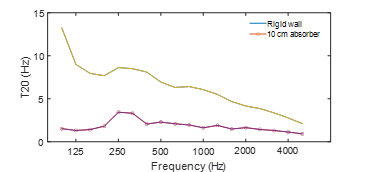Figure 2. Reverberation time with and without a porous absorber in a rectangular room .

### 2. Simulation with Treble​

Treble is a hybrid room acosutic simulation with discontinuous Galerkin finite element method, DGFEM, at lower frequencies and GA at higher frequencies. It has been proven that DGFEM can accuately simulate the sound field by solving the wave equation with accurate boundary conditions . The transition frequency for the following simulation is 1414 Hz, so the results up to the 1 kHz octave band are obtained by DGFEM and the 2k and 4kHz octave band resuts are simulated by the GA simulation engine.

### 2.1. Porous Materials​

Two materials are used: Ecophon Akusto™ Wall A, 4 cm, with an air flow resistivity of 47 kPa∙s/m2 and Ecophon Industry™ Modus, 10 cm, with an air flow resistivity of 11 kPa∙s/m2. Industry Modus has higher absorption coefficients than Akusto Wall A, as can be seen in Fig. 3.Figure 3. Theoretical random incidence absorption coefficient of Ecophon Akusto wall A and Industry Modus. For more info about the absorbers, see https://www.ecophon.com/en/products/wall-applications/akusto/akusto-wall-a2/#Acoustics and https://www.ecophon.com/dk/ecophon/modular-ceilings/industry/industry-modus/.

### 2.2. Porous material modeling​

To calculate the surface impedance with the flow resistivity known, Miki’s impedance model was used to calculate the surface impedance of the test absorbers, which reads 

$Z_c = \rho_0 c_0 \left( 1 + 0.070 \left( \frac{f}{\sigma} \right)^{-0.632} - j\,0.107 \left( \frac{f}{\sigma} \right)^{-0.632} \right)$
$k_t = \frac{\omega}{c_0} \left( 1 + 0.109 \left( \frac{f}{\sigma} \right)^{-0.618} - j\,0.160 \left( \frac{f}{\sigma} \right)^{-0.618} \right)$

Based on Zc and kt, the surface impedance can be computed. For an absorber having a thickness d, which has a backing surface impedance of Z|x=d, the surface impedance in front of the entire construction at x = 0 for a plane wave incident at an angle θ is calculated by 

$Z_w (f,θ)=\frac{Z_c k_o}{k_x} \left[\frac{(-jZ|_{x=d} \cot( k_x d)+Z_c \frac{k_o}{k_x} )}{(Z|_{x=d}-jZ_c \frac{k_o}{k_x} \cot( k_x d))}\right]$

where kx is the normal component of kt, ko is the wave number in air, and j is the imaginary unit. If the absorber is rigidly backed (Z|x=d = ∞), Eq. (7) becomes . For absorbers backed by an air cavity, the backing impedance = , where do is the air cavity depth. The corresponding normal-incidence pressure reflection coefficient is calculated as follows:

$R_p (f,\theta_i )=\frac{Z_w (f,\theta_i ) \cos \theta_i -\rho_o c_o}{Z_w (f,\theta_i ) \cos \theta_i +\rho_o c_o }$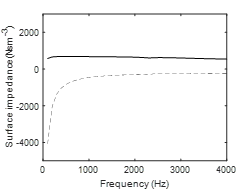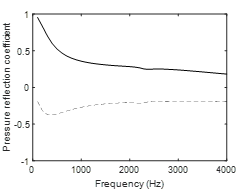Figure 4. Surface impedance and pressure reflection coefficient of Akusto wall A via Miki’s model.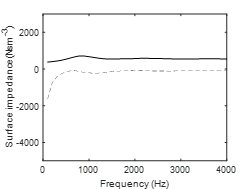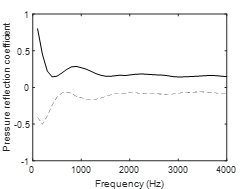Figure 5. Surface impedance and pressure reflection coefficient of Industry Modus via Miki’s model.

It is noted that the calculated absorption coefficient assuming uniform sound incidence onto the specimen (termed random incidence absorption coefficient) is not the same as the measured sound absorption coefficient via ISO 352 in reverberation chambers . Here is an example of Akusto wall A, which shows that the measured (and later simplified) practical absorption coefficient is always higher than the calculated random incidence absorption coefficient.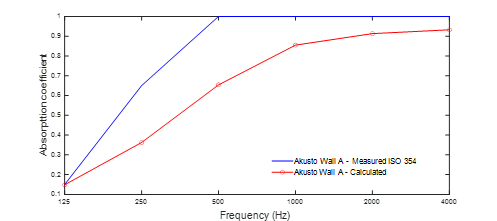Figure 6. Practical absorption coefficient and random incidence absorption coefficient calculated for Akusto Wall A.

### 2.3. Rectangular room​

A small cuboid with dimensions of 4.38 × 3.27 × 3.29 [all in m in what follows] was measured by Thydal with and without a porous ceiling . As mentioned, 4 cm Akusto Wall A and 10 cm thick Industry Modus absorbers are used.Figure 7. DTU room with measurement setup (Dirac software version 6.0, onmi-directional sound source, omni-directional B&K Type 4192 microphone)  and simulation screenshot. Source 1 (3.96, 0.42, 1.6), Source 2 (1.8, 2.25, 1.45), Receiver 1 (1.3, 1.4, 1.45), Receiver 2 (2.78, 1, 1.6).

## 3. Results​

### 3.1. Transfer function (TF) comparison​

Two TFs are compared below 500 Hz in Fig. 8. In both cases, excellent agreement is made. The eigen-frequencies agree well, which are also called the room modes and natural frequencies. This shows that Treble can account for interference by including the phase information. This is delightful news for room acoustic simulation software users, as no energy-based GA can do such accurate simulations in terms of TF calculations.Figure 8. TF comparison between measurement and simulation. S1R1 with Ecophon Industry Modus (left) and S2R2 with Ecophon Akusto Wall A (right) .

### 3.2. Room acoustic parameters ISO 3382-1​

The room acoustic parameters (ISO 3382-1 ) with Akusto Wall A are calculated via Dirac version 6.0 (B&K type 7841). Two reverberation time measures, EDT and T20, and D50 and center time (Ts) are shown. Averaged over the 4 source-receiver combinations, they agree very well. Particularly the T20 has a low value at around 200 Hz, while a peak is observed at around 320 – 500 Hz from the measurement and simulation. This indicates the Treble simulation captures the transition from the 3D sound field to the 2D sound field well. In the mid frequency between 250 and 2000 Hz, T20 is significantly higher than EDT, meaning a double sloped decay trend in this frequency range. Particularly EDT agrees very well, meaning the initial decay is quite accurately predicted by the treble simulation, including such complicated two peaks around 400 Hz and 800 Hz.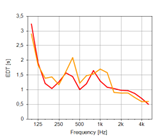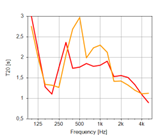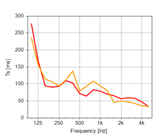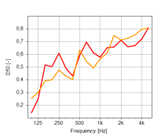A similar agreement is found in the following figures about Modus. The reverberation time at 125 Hz is lowest due to a 3D sound field behavior where the decay rate is much faster than in 2D. However, the sound field becomes more towards 2D at 300 Hz and beyond, where the sound absorption from the Industry Modus absorber dominates, which leads to a longer decay over the grazing directions to the absorber surface.Figure 10. Room acoustic parameters with Industry Modus. Dark green: measurements, Light green: Treble simulations.

Figure 11 explains why such an undulation in the reverberation time curve happens in empty rooms with a porous layer. Skålevik and Vennerød called it ‘a hard case’, indicating that a highly absorbing surface on one surface, typically the ceiling, makes the sound fields behaving like a 2D. One can observe double-sloped energy decays, where a fast initial decay is followed by a much gentler slope of energy decay due to a grazing mode . A much shorter EDT than T20 shown in Figs. 9 and 10 is an indication of the 2D sound field. In such a 2D sound field due to the fast-decaying vertical propagation, the grazing modes dominates and accordingly the reverberation time such as T20 and T30 increases at frequencies where a strong 2D behavior exhibits. At such frequencies, the Sabine theory does not hold and underestimates the reverberation time. This also happens with energy-based GA simulations when the random incidence absorption coefficient is assumed to hold for all incidence angles.Figure 11. Reverberation time in hard cases (left) and double-sloped energy decay (right) .

In Fig. 12, an example of the double-sloped decay is shown. The energy decay curve (EDT) at the 500 Hz octave band with Akusto wall A is shown, where T20 is substantially increased compared to EDT. On the right, Nilsson’s well known curved decay example in Ref.  is shown. The dotted line on Nilsson’s example is a predicted decay using the Sabine’s formula, i.e., 3D sound field, which differs from the measurement after about 100 ms from the direct sound.Figure 12. Double-sloped decay with Akusto wall A at 500 Hz by Treble simulation over the 1.8 s (left) and double sloped decay at 1000 Hz in Nilsson’s measurement .

## 4. Conclusions​

This study investigates how a rectangular room can behave as a 2D-like sound field when a porous absorber is concentrated on a single wall. With the 2D behavior, the measured reverberation time curve is undulated, exhibiting an unexpected high reverberation time at mid frequency range. These frequencies with the increased reverberation time are linked to the degree of inhomogeneity of the boundary absorption. Treble, as a hybrid room acoustic simulation solver that uses a wave-based method for low frequencies, can effectively replicate such 2D behavior and help acousticians analyze such tricky yet quite common cases.

 G. Marbjerg, J. Brunskog, C.-H. Jeong: The difficulties of simulating the acoustics of an empty rectangular room with an absorbing ceiling, Applied Acoustics, 141, 2018, 35-45.

 M. Tohyama and H. Suzuki: Reveberation time in an almost-two-diemnsioanl diffuse field. J. Sound Vib. 111, 1986, 391-398.

 J. Vennerød: The Hard Case - Improving Room Acoustics in Cuboid Rooms by using Diffusors, Report from NTNU, 2013.

 M. Skålevik: How much scattering is sufficient tao soften the hard case?, Forum Acusticum, Krakow, Poland, 2014.

 T. Thydal, F. Pind, C.-H. Jeong, A. P. Engsig-Karup: Experimental validation and uncertainty quantification in wave-based computational room acoustics, Applied Acoustics, 178, 2021, 107939.

 Y. Miki: Acoustical properties of porous materials - modifications of Delany-Bazley models. J. Acoust. Soc. Jp. 11, 1990, 19-28.

 J.-F. Allard: Propagation of Sound in Porous Media: Modelling Sound Absorbing materials. Elsevier Applied Science, London, 1993.

 ISO 354: Acoustics - Measurement of sound absorption in a reverberation room. 2003.

 ISO 3382-1: Acoustics - Measurement of room acoustic parameters — Part 1: Performance spaces. 2009.

 E. Nilsson: Decay processes in rooms with non-diffuse sound fields. Part I: ceiling treatment with absorbing material. Building acoustics, 11, 2004, 39-60.